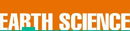# Newton, Sir Isaac (1642-1727) - Research Article from World of Earth Science

This encyclopedia article consists of approximately 3 pages of information about Newton, Sir Isaac (1642-1727).
 This section contains 738 words (approx. 3 pages at 300 words per page) View a FREE sampleEnglish physicist

In 1687, English physicist Sir Isaac Newton published a law of universal gravitation in his important and profoundly influential work Philosophiae Naturalis Principia Mathematica (Mathematical principles of natural philosophy). Newton articulated a law of universal gravitation that states that bodies with mass attract each other with a force that varies directly as the product of their masses and inversely as the square of the distance between them. This mathematically elegant law, along with Newton's laws of motion, became the guiding models for the future development of physical law.

Newton admitted having no fundamental explanation for mechanism of gravity itself. In Principia Newton stated, "I have been unable to discover the cause of those properties of gravity from phenomena, and I feign no hypotheses" (regarding its mechanism). Moreover, Newton asserted, "To us it is enough that gravity does really exist, and act...

 This section contains 738 words (approx. 3 pages at 300 words per page) View a FREE sample